# NCERT Solutions for Class 8 Maths Chapter 3 - Understanding Quadrilaterals

## Q1 Take any quadrilateral, say ABCD (Fig 3.4). Divide it into two triangles, by drawing a diagonal. You get six angles 1, 2, 3, 4, 5 and 6. Use the angle-sum property of a triangle and argue how the sum of the measures of and amounts to .As shown in ACD,

As shown in ABC,

( Since,  , ,,

)

Hence proved,  the sum of the measures of and
amounts to .What can you say about the sum of the angles ∠1, ∠2, ∠3 and ∠4?

[Note: We denote the angles by ∠1, ∠2, ∠3, etc., and their respective measures by m∠1, m∠2, m∠3, etc.]

The sum of the measures of the four angles of a quadrilateral is___________.
You may arrive at this result in several other ways also.

As we know the sum of all four angles of a quadrilateral is.

are four angles of quadrilateral ABCD.

Hence, the sum of these angles is  =

Angles ∠1, ∠2, ∠3, ∠4 meet at a point and sum of these angles is  = .As we know

Hence, sum of .Draw a line matching points B and D.Line BD divides ABCD in two triangles . and .

Sum of angles of  =

Sum of angles of  =

Here,

Adding equation 1 and equation 2,

{    and

i.e.  = .                                                                                     }

The sum of the interior angles in a quadilateral,  = .

## NCERT solutions for class 8 maths chapter 3 Understanding Quadrilaterals Excercise: 3.1Classify each of them on the basis of the following.

(a) Simple curve

(a) Simple curve : The curve which does not cross itself and have only one curve.

Some simple curves areClassify each of them on the basis of the following.

(b) Simple closed curve

Simple closed curve : The simple curves which are closed by line segment or curved line.

Some simple closed curve areClassify each of them on the basis of the following.

(c) Polygon

A normally closed curve made up of more than 4 line segments is called a polygon.

Some polygons are shown in figuresClassify each of them on the basis of the following.

(d) Convex polygon

(d) Convex polygon :Convex polygon are polygons having all interior angles less than .

Convex polygon = 2.Classify each of them on the basis of the following.

(e) Concave polygon

(e) Concave polygon : Concave polygon have one or more interior angles greater than .

Concave polygon = 1.

Q2 (a) How many diagonals does each of the following have?There are 2 diagonals in a convex quadrilateral.

A regular hexagon

(b) A regular hexagon :Total number of diagonal a regular hexagon have are 9 .

A triangle

(c) A triangleA triangle does not have any diagonal.

The sum of the measures of the angles of a convex quadrilateral is . A convex quadrilateral is made of two triangles and sum of angles of a triangle is . Hence,sum of angles of two triangles is  which is also sum of angles of a quadrilateral.Here,as we can see this quadrilateral has two triangles and the sum of angles of these triangles is = sum of all angles of a quadrilateral.What can you say about the angle sum of a convex polygon with number of sides?

(a) 7

(a) 7

the sum of angles of a convex polygon

the sum of angle of a convex polygon with 7 sides

the sum of angles of a convex polygon with 7 sides

the sum of angles of a convex polygon with 7 sidesWhat can you say about the angle sum of a convex polygon with the number of sides?

(b) 8

(b) 8

sum of the angles of a convex polygon

sum of the angles of a convex polygon with 8 sides

sum of the angles of a convex polygon with 8 sides

sum of the angles of a convex polygon with 8 sidesWhat can you say about the angle sum of a convex polygon with number of sides?

(c) 10

(c) 10

angle sum of a convex polygon

angle sum of a convex polygon with 10 sides

angle sum of a convex polygon with 10 sides

angle sum of a convex polygon with 10 sidesWhat can you say about the angle sum of a convex polygon with number of sides?

(d)

(d)

All the angles of a convex polygon added up to

the sum of the angles of a convex polygon having n sides

A regular polygon is a polygon which has equal sides and equal angles.

The name of a regular polygon of 3 sides is an equilateral triangle.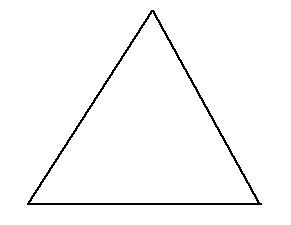All sides of the equilateral triangle are equal and angles are also equal.

Each angle =

A regular polygon is a polygon which has equal sides and equal angles.

The name of a regular polygon of 4 sides is square.Square has all angles of and all sides are equal.

A regular polygon is a polygon which have equal sides and equal angles.

The name of a regular polygon of 6 sides is a hexagon.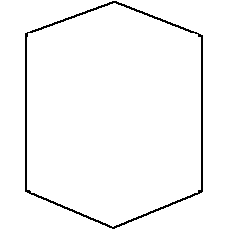All angles of the hexagon are  each.The solution for the above-written question is mentioned below,

Sum of angles of a quadrilateralThe Solution for the above-written question is mentioned below,

Sum of angles of a quadrilateralThis pentagon has all sides and all angles equal.

Sum of all angles of pentagon is(linear pair)                                                                  (linear pair)

Sum of all angles of pentagon =

Q7 (a) Find(a) FindSum of all angles of triangle is

the unmarked angle of triangle be A.

=

=

Q7 (b) FindHere you will find the detailed solution of the above-written question,Sum of all angles of quadrilateral =

Let the unmarked angle be A.

=

=

## NCERT solutions for class 8 maths chapter 3 Understanding Quadrilaterals Excercise: Sum of the Measures of the Exterior Angles of a Polygon

Take a regular hexagon Fig 3.10.
Q1 What is the sum of the measures of its exterior anglesthe sum of all its exterior angles will be equal to .

x,y,z,p,q,r are all exterior angles.

Hence,

Is . Why?It is a hexagon with all sides equal.

All interior angles are also equal.

(linear pairs)

Hence,

because it is a hexagon with all sides and angles equal.It is a hexagon with all sides equal.

All interior angles are also equal.

Sum of all angles of hexagon is .

(linear pairs)Each exterior angle =  =It is a hexagon with all sides equal.

All interior angles are also equal.

Sum of all angles of hexagon is .(i) a regular octagon: It has all 8 angles equal and sum of all eight angles is 1080. Interior angles are equal so exterior angles are also equal.

Let interior angle be A.

.

All exterior angles be B.

(ii) a regular 20-gon: It has all 20 angles equal and sum of all eight angles is 3240.Interior angles are equal so exterior angles are also equal.

Let interior angle be A.

.

All exterior angles be B.

## NCERT solutions for class 8 maths chapter 3 Understanding Quadrilaterals Excercise: 3.2Sum of all exterior angles of polygon isSum of all exterior angles of polygon is

A regular polygon of 9 sides have all sides,interior angles and exterior angles equal.

Sum of exterior angles of a polygon =

Let interior angle be A.

Sum of exterior angles of 9 sided polygon =

Exterior angles of 9 sided polygon

Hence, measure of each exterior angle of a regular polygon of 9 sides is

A regular polygon of 15 sides have all sides,interior angles and exterior angles equal.

Sum of exterior angles of a polygon =

Let interior angle be A.

Sum of exterior angles of 15 sided polygon =

Exterior angles of 15 sided polygon

Hence, measure of each exterior angle of a regular polygon of 15 sides is

The measure of an exterior angle is 24°

Regular polygon has all exterior angles equal.

Sum of exterior angles of a polygon =

Let number of sides be X.

Sum of exterior angles of a polygon =

Exterior angles of 15 sided polygon

Hence,  sided  regular polygon have measure of an exterior angle  24°

The measure of each interior angle is 165°

So, measure of each exterior angle = 180°-165° = 15°

Regular polygon has all exterior angles equal.

Let number of sides of polygon = n

Sum of Exterior angles of a polygon =

Hence,regular polygon having each of its interior angles is 165° has 24 sides.

The measure of an exterior angle is 22°

Regular polygon has all exterior angles equal.

Sum of exterior angles of a polygon =

Let number of sides be X.

Sum of exterior angles of a polygon =

Exterior angles of 15 sided polygon

Hence,side of a polygon should be an integer but as shown above side is not a integer.So,it is not  possible to have a regular polygon with measure of each exterior angle as 22 .

The measure of an interior angle is 22°

Regular polygon has all interior angles equal.

Let number of sides and number of interior angles be n.

Sum of interior angles of a polygon =

Sum of interior angles of a polygon =  =

Number of sides of a polygon should be an integer but since it is not an integer.So, it cannot be a regular polygon with interior angle as 22

Q6 (a) What is the minimum interior angle possible for a regular polygon? Why?

Consider a polygon with the lowest number of sides i.e. 3.

Sum of interior angles of 3 sided polygon =

Interior angles of regular polygon are equal = A .

Hence,the minimum interior angle possible for a regular polygon is .

Let there be  a polygon with minimum number of sides i.e. 3.

Exterior angles a equilateral triangle has maximum measure.

Sum of exterior angles of polygon =

Let exterior angle be A.

Hence,the maximum exterior angle possible for a regular polygon is .

## Solutions of NCERT for class 8 maths chapter 3 Understanding Quadrilaterals Topic: Kinds of QuadrilateralsYou get a trapezium. (Check it!) Which are the parallel sides here? Should the
non-parallel sides be equal?
You can get two more trapeziums using the same set of triangles. Find them out and
discuss their shapes.AB and CD are parallel sides.BC and AD are nonparallel sides. Non parallel sides need not be equal.

We can get two more trapeziums using the same set of triangles-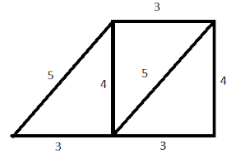and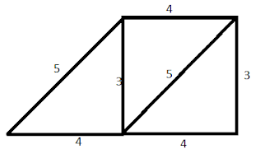## NCERT solutions for class 8 maths chapter 3 Understanding Quadrilaterals Topic: Kite

 Show that and are congruent. What do we infer from this?Fold both the diagonals of the kite. Use the set-square to check if they cut at
right angles. Are the diagonals equal in length?
Verify (by paper-folding or measurement) if the diagonals bisect each other.
By folding an angle of the kite on its opposite, check for angles of equal measure.
Observe the diagonal folds; do they indicate any diagonal being an angle bisector?
Share your findings with others and list them. A summary of these results are
given elsewhere in the chapter for your reference.Kite has symmetry along AC diagonal. and  are congruent and equal triangles.

Diagonals AC and BD are of different lengths.

Diagonals bisect each other.

The two diagonals AC and BD bisect .

## NCERT solutions for class 8 maths 3 Understanding Quadrilaterals Excercise: Elements of ParallelogramHere is same as except for the name. Similarly the other corresponding
sides are equal too.
Place over . Do they coincide? What can you now say about the lengths
and ?
Similarly examine the lengths and . What do you find?
You may also arrive at this result by measuring and .Take cut-outs of two identical parallelograms, say ABCD and A′B′C′D′ (Fig 3.19).

Here is same as .

Also, is same as

Place over . They coincide with each other.

The lengths  and  are equal and parallel lines.

The lengths and  are equal and parallel lines.

Property: The opposite sides of a parallelogram are of equal length.Property : The opposite sides of a parallelogram are of equal length.

As we can see in the figure above, the opposite sides of figure are equal.

The figure above is a rectangle which is part of parallelogram.

Hence, the opposite sides of a parallelogram are of equal length.

Property: The opposite angles of a parallelogram are of equal measure.As shown in the above figure opposites angles are equal and are equal to .

Hence,the figure obtained help you to confirm the property: The opposite angles of a parallelogram are of equal measure.ABCD (Fig 3.29). Let its diagonals and meet at O.
Find the mid point of by a fold, placing C on A. Is the
mid-point same as O?
Does this show that diagonal bisects the diagonal at the point O? Discuss it
with your friends. Repeat the activity to find where the mid point of could lie.ABCD . Let its diagonals and meet at O.
The mid point of  is at point O.Hence, the
mid-point is same as O.

This show that diagonal bisects the diagonal at the point O.

The mid point of  is point O.

## NCERT solutions for class 8 maths chapter 3 Understanding Quadrilaterals Excercise: 3.3......

In a parallelogram, opposite sides are equal in lengths.Hence,......

In a parallelogram ,opposite angles are equal.(ii)......

In a parallelogram, both diagonals bisect each other......

In a parallelogram, adjacent angles are supplementary to each other.(iv)In a parallelogram, adjacent angles are supplementary to each other.

Opposite angles are equal.

Hence, z =

and y = 100( Two adjacent angles are supplementary to each other)

x=y=                 (opposite angles are equal)

z=x=              ( corresponding angles are equal)x=                 (vertically opposite angles)

(sum of angles of a triangle is )

y=z=                (alternate interior angles)y =                    ( opposite angles are equal)

z=                     (corresponding angles are equal)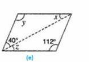y =                                       (opposite angles are equal)

x = z =            (alternate angles are equal)

(i)

Opposite angles should be equal and adjacent angles should be supplementary to each other.

are opposite angles.

Hence, a quadrilateral ABCD can be a parallelogram but it is not confirmed.

and ?

Opposite sides of a parallelogram are equal in length.

Since,   and  are opposite sides and have different lengths.

No, it is not a parallelogram.

Opposite angles of a parallelogram are equal

Since here   and  are different.

So, it is not a parallelogram.The above-shown figure shows two opposite angles equal..

But, it's not a parallelogram because the other two angles are different i.e. .

The measures of two adjacent angles of a parallelogram are in the ratio 3 : 2.

Sum of adjacent angles is .

Hence, angles are  and .

Let there be a parallelogram ABCD then,        and . (Opposite angles are equal).

Given: Two adjacent angles of a parallelogram have equal measure = .

( adjacent angles of a parallelogram are supplementary)

and      ( Opposite angles of a parallelogram are equal)

Hence,The adjacent figure HOPE is a parallelogram.

(linear pairs)

(opposite angles of a parallelogram are equal)

( adjacent angles are supplementary )

y=                        (Alternate interior angles are equal)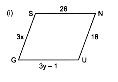GUNS is a parallelogram, so opposite sides are equal in length

Hence, cm  and   cm.Diagonals of a parallelogram intersect each other.Hence,cm  and   cm.(oppsite angles are equal)

(sum of angles of a triangle is )Given, .

A transverse line is intersecting two lines such that the sum of angles on the same side of the transversal line is .

And hence, lines KL and MN are parallel to each other.

Quadrilateral KLMN has a pair of parallel lines so it is a trapezium.Given ,(Angles on same side of transversal)

Hence,.Given,

(angles on same side of transversal)

(angles on same side of transversal)

Yes, to find there is more than one method.

PQRS is quadrilateral so the sum of all angles is 360

and we know

so put values of  and we get a measurement of

## NCERT solutions for class 8 maths chapter 3 Understanding Quadrilaterals Topic: Squares

Property: The diagonals of a square are perpendicular bisectors of each other.PO = OR       ( Square is a type of parallelogram)

By SSS congruency rule,    and   are congruent triangles.

&

Hence,.

Thus,we can say that the diagonals of a square are perpendicular bisectors of each other

## NCERT solutions for class 8 maths 3 Understanding Quadrilaterals Excercise: 3.4

(a). False, All squares are rectangle but all rectangles cannot be square.

True.Opposite sides of the rhombus are parallel and equal.

True. All squares are rhombus because rhombus has opposite sides parallel and equal and same square has.

Also all squares are rectangles because they have all interior angles as .

False.

All squares have there opposite sides equal and parallel .Hence,they are parallelogram.

False,

Kites do not have all sides equal so they are not a rhombus.

True,all rhombuses are kites because they have two adjacent sides equal.

True,all parallelograms are trapezium because they have a pair of parallel sides.

True,All squares are trapeziums because all squares have pair of parallel sides.

The quadrilateral having  four sides of equal length are square and rhombus.

All the quadrilaterals that have four right angles are rectangle and square

A square is a quadrilateral because square has four sides.

A square is a parallelogram because square has opposite sides parallel to each other.

A square is a rhombus because square has four sides equal.

A square is a rectangle sinse it has all interior angles of .

The quadrilaterals whose diagonals bisect each other are square, rectangle, parallelogram and rhombus.

The quadrilaterals whose diagonals are perpendicular bisectors of each other are rhombus and square.

The quadrilaterals whose diagonals are equal are squares and rectangles.

A rectangle is a convex quadrilateral because it has two diagonals and both lie in the interior of the rectangle.Draw line AD and DC such that  and .

and

ABCD is a rectangle as it has opposite sides equal and parallel.

All angles of the rectangle are  and a rectangle has two diagonals equal and bisect each other.

Hence, AO = BO = CO = DO

O is equidistant from A,B,C,D.

## Solutions of NCERT for class 8 maths chapter 3 Understanding Quadrilaterals Topic: Rectangle

(1).All the properties of a parallelogram.

(2) Each of the angles is a right angle.

(3) Diagonals are equal

## Q2 A square was defined as a rectangle with all sides equal. Can we define it as

rhombus with equal angles? Explore this idea.

Properties of rectangle are :

(1) All the properties of a parallelogram.

(2) Each of the angles is a right angle.

(3) Diagonals are equal.

A square satisfies all the properties of rectangles so a square can be defined as a rectangle with all sides equal.

Properties of rhombus are :

(1) All the properties of a parallelogram.

(2) Diagonals are perpendicular to each other.

A square satisfies all the properties of rhombus so we can define it as
rhombus with equal angles.

## Solutions of NCERT for class 8 maths chapter 3 Understanding Quadrilaterals Topic: Trapezium

Trapezium has two sides parallel and other two sides are non parallel.Parallel sides may be equal or unequal but we cannot have a trapezium with all sides and angles equal.

## NCERT solutions for class 8 maths: Chapter-wise

 Chapter -1 NCERT solutions for class 8 maths chapter 1 Rational Numbers Chapter -2 Solutions of NCERT for class 8 maths chapter 2 Linear Equations in One Variable Chapter-3 CBSE NCERT solutions for class 8 maths chapter 3 Understanding Quadrilaterals Chapter-4 NCERT solutions for class 8 maths chapter 4 Practical Geometry Chapter-5 Solutions of NCERT for class 8 maths chapter 5 Data Handling Chapter-6 CBSE NCERT solutions for class 8 maths chapter 6 Squares and Square Roots Chapter-7 NCERT solutions for class 8 maths chapter 7 Cubes and Cube Roots Chapter-8 Solutions of NCERT for class 8 maths chapter 8 Comparing Quantities Chapter-9 NCERT solutions for class 8 maths chapter 9 Algebraic Expressions and Identities Chapter-10 CBSE NCERT solutions for class 8 maths chapter 10 Visualizing Solid Shapes Chapter-11 NCERT solutions for class 8 maths chapter 11 Mensuration Chapter-12 NCERT Solutions for class 8 maths chapter 12 Exponents and Powers Chapter-13 CBSE NCERT solutions for class 8 maths chapter 13 Direct and Inverse Proportions Chapter-14 NCERT solutions for class 8 maths chapter 14 Factorization Chapter-15 Solutions of NCERT for class 8 maths chapter 15 Introduction to Graphs Chapter-16 CBSE NCERT solutions for class 8 maths chapter 16 Playing with Numbers

## How to use NCERT solutions for class 8 maths chapter 3 Understanding Quadrilaterals?

• Learn how to use their properties in specific questions using the solved examples.
• Practice the problems given in the NCERT textbook.
• During the practice, if you find a problem in solving any of the questions you can use NCERT solutions for class 8 maths chapter 3 Understanding Quadrilaterals.

Keep working hard and happy learning!